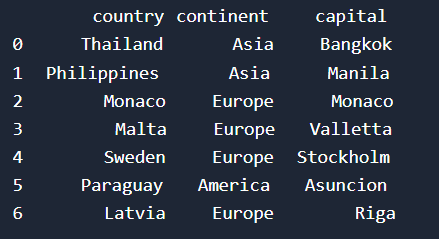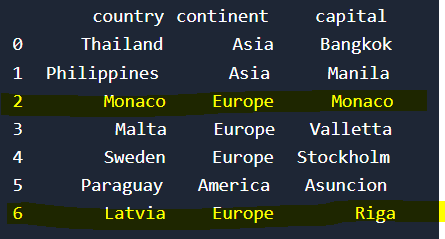Course Content

Pandas First Steps

##Function iloc() 1/2

We can also access data by index in the dataframe. Accessing rows by index is possible in several ways:

• `.iloc` - used to access by numeric value (starting from 0).
• `.loc` - used to access by string label.

In this course, we will get acquainted with the `.iloc()` function only.

Let's create a dataframe that we will work with.This dataframe has the following form:Here we see the first column, which is responsible for indexing rows. Using these indexes, we will access the cells from the dataframe. Let's take a look the syntax of this function:

It's time to use this function on our dataframe.If we run the code above, we will get the rows that are marked on the picture below:Everything was clear?

Section 1. Chapter 13